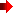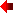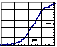Monoprotic Buffers (lig)

The titration of a monoprotic bufferserves as a model for monovalent ligand binding systems in general. In the example outlined below, protonated ("saturated") acetic acid undergoes a one-step dissociation (ionization) reaction converting to the deprotonated ("desaturated") acetate in proportion to decreasing hydronium ion concentration. In the reverse direction, acetate undergoes a one-step association reaction forming acetic acid in proportion to increasing hydronium ion concentration.

One-step dissociationof acetic acidstep1d
CH3-COOH
 - H++ H+
CH3-COO-
step1aOne-step association
of acetate
 Kd-cooh = [ H+] * [CH3-COO-] / [CH3-COOH]step 1d Ka-cooh = [CH3-COOH] / [ H+] * [CH3-COO-]step 1a

Quantitatively, this monovalent ligand binding system can be described in terms of Ya, the "saturation (association) fraction:"

Ya=[HAc] / ( [ Ac- ] + [ HAc ] ) =[HAc] / Co
Ya=[H+] / ( [H+] + Kd )

where Co = [Ac- ] + [ HAc ] and Kd = [H+] * [ Ac- ] / [ HAc ] .Prove:

1. if Ya = 0.5, [H+]50%=Kd, the H+ concentration at 50% saturation;
2. Ya=Ka * [H+] / ( Ka * [H+] + 1 )
where
Ka= [ HAc ] / ( [H+] * [ Ac- ] )

Yd is defined as the "dissociated fraction," that is, the total percentage of molecules in the carboxylate form. By definition, Yd= 1 - YaProve:

1. Yd= [Ac-] / Co

2. Yd=[Ac-] / ( [ Ac- ] + [ HAc ] )

3. Yd=Kd / ( [H+] + Kd )

4. Yd=1 / ( Ka * [H+] + 1 )

In a biological system the saturation fraction can be a dynamic parameter, changing as the organism responds to chemical changes in the environment. For example, the saturation fraction of hemoglobin in terms of bound oxygen changes as the molecules course through the blood moving from areas of high oxygen concentration (lungs) to areas of low oxygen concentration (capillaries). The changes in hemoglobin saturation fraction account for the delivery of oxygen to tissues that are respiring aerobically. A couple of ways to think about the saturation fraction is that this parameter is akin to a sponge continuously being squeezed and soaked, or a glass continuously being filled and emptied. The dyanmics of the saturation fraction are animated in a cartoon of wine glass being filled and emptied.

Continue the acid-base titration analysis of acetic acid/acetate by opening the Excel spreadsheet and following the directions found there. You will find four types of saturation graphs showing

• Ya, the associated fraction, plotted against [H+] (Rectangular Hyperbolic Plot);
• Ya plotted against pH ( i.e., -log [H+] ) (pH Saturation Plot);
• Ya plotted against plotted against [H+] on a log axis (Log Saturation Plot);
• log (Yd / Ya ) plotted against plotted against pH ( i.e., -log [H+] ) (Henderson-Hasselbalch Plot).

Answer the questions on the "Questions worksheet, the last of the series.

Acid-Base Titration of Acetic Acid:Monovalent Ligand BindingReturn to Biochemistry 108A Home PagePress the browser's "Back" key to return to previous page.Search for a Specific Topic